# 19  Case Study in Parametric Survival Modeling and Model Approximation

Data source: Random sample of 1000 patients from Phases I & II of SUPPORT (Study to Understand Prognoses Preferences Outcomes and Risks of Treatment, funded by the Robert Wood Johnson Foundation). See Knaus et al. (1995). The dataset is available from hbiostat.org/data.

• Analyze acute disease subset of SUPPORT (acute respiratory failure, multiple organ system failure, coma) — the shape of the survival curves is different between acute and chronic disease categories
• Patients had to survive until day 3 of the study to qualify
• Baseline physiologic variables measured during day 3
A

## 19.1 Descriptive Statistics

Create a variable acute to flag categories of interest; print univariable descriptive statistics.

Code
require(rms)
options(prType='html')     # for print, summary, anova
getHdata(support)          # Get data frame from web site
acute <- support$dzclass %in% c('ARF/MOSF','Coma') des <- describe(support[acute,]) sparkline::sparkline(0) # load sparkline dependencies Code maketabs(print(des, 'both'), wide=TRUE, initblank=TRUE) Variable Label n Missing Distinct Info Mean Gini |Δ| support[acute, ] Descriptives 24 Continous Variables of 35 Variables, 537 Observations age Age 537 0 529 1.000 60.7 19.98 28.49 35.22 47.93 63.67 74.49 81.54 85.56 slos Days from Study Entry to Discharge 537 0 85 0.999 23.44 22.24 4.0 5.0 9.0 15.0 27.0 47.4 68.2 d.time Days of Follow-Up 537 0 340 1.000 446.1 566.1 4 6 16 182 724 1421 1742 edu Years of Education 411 126 22 0.957 12.03 3.581 7 8 10 12 14 16 17 scoma SUPPORT Coma Score based on Glasgow D3 537 0 11 0.822 19.24 27.87 0 0 0 0 37 55 100 charges Hospital Charges 517 20 516 1.000 86652 90079 11075 15180 27389 51079 100904 205562 283411 totcst Total RCC cost 471 66 471 1.000 46360 46195 6359 8449 15412 29308 57028 108927 141569 totmcst Total micro-cost 331 206 328 1.000 39022 36200 6131 8283 14415 26323 54102 87495 111920 avtisst Average TISS, Days 3-25 536 1 205 1.000 29.83 14.19 12.46 14.50 19.62 28.00 39.00 47.17 50.37 meanbp Mean Arterial Blood Pressure Day 3 537 0 109 1.000 83.28 35 41.8 49.0 59.0 73.0 111.0 124.4 135.0 wblc White Blood Cell Count Day 3 532 5 241 1.000 14.1 9.984 0.8999 4.5000 7.9749 12.3984 18.1992 25.1891 30.1873 hrt Heart Rate Day 3 537 0 111 0.999 105 38.59 51 60 75 111 126 140 155 resp Respiration Rate Day 3 537 0 45 0.997 23.72 12.65 8 10 12 24 32 39 40 temp Temperature (celcius) Day 3 537 0 61 0.999 37.52 1.505 35.50 35.80 36.40 37.80 38.50 39.09 39.50 pafi PaO2/(.01*FiO2) Day 3 500 37 357 1.000 227.2 125 86.99 105.08 137.88 202.56 290.00 390.49 433.31 alb Serum Albumin Day 3 346 191 34 0.997 2.668 0.7219 1.700 1.900 2.225 2.600 3.100 3.400 3.800 bili Bilirubin Day 3 386 151 88 0.997 2.678 3.507 0.3000 0.4000 0.6000 0.8999 2.0000 6.5996 13.1743 crea Serum creatinine Day 3 537 0 84 0.998 2.232 1.997 0.6000 0.7000 0.8999 1.3999 2.5996 5.2395 7.3197 sod Serum sodium Day 3 537 0 38 0.997 138.1 7.471 129 131 134 137 142 147 150 ph Serum pH (arterial) Day 3 500 37 49 0.998 7.416 0.08775 7.270 7.319 7.380 7.420 7.470 7.510 7.529 glucose Glucose Day 3 297 240 179 1.000 167.7 92.13 76.0 89.0 106.0 141.0 200.0 292.4 347.2 bun BUN Day 3 304 233 100 1.000 38.91 31.12 8.00 11.00 16.75 30.00 56.00 79.70 100.70 urine Urine Output Day 3 303 234 262 1.000 2095 1579 20.3 364.0 1156.5 1870.0 2795.0 4008.6 4817.5 adlsc Imputed ADL Calibrated to Surrogate 537 0 144 0.956 2.119 2.386 0.000 0.000 0.000 1.839 3.375 6.000 6.000 Variable Label n Missing Distinct Info Sum Mean Gini |Δ| support[acute, ] Descriptives 11 Categorical Variables of 35 Variables, 537 Observations death Death at any time up to NDI date:31DEC94 537 0 2 0.670 356 0.6629 0.4477 sex 537 0 2 hospdead Death in Hospital 537 0 2 0.703 201 0.3743 0.4693 dzgroup 537 0 3 dzclass 537 0 2 num.co number of comorbidities 537 0 7 0.926 1.525 1.346 income 335 202 4 race 535 2 5 adlp ADL Patient Day 3 104 433 8 0.875 1.577 2.152 adls ADL Surrogate Day 3 392 145 8 0.888 1.86 2.466 sfdm2 468 69 5 B Code spar(ps=11) # Show patterns of missing data plot(naclus(support[acute,]))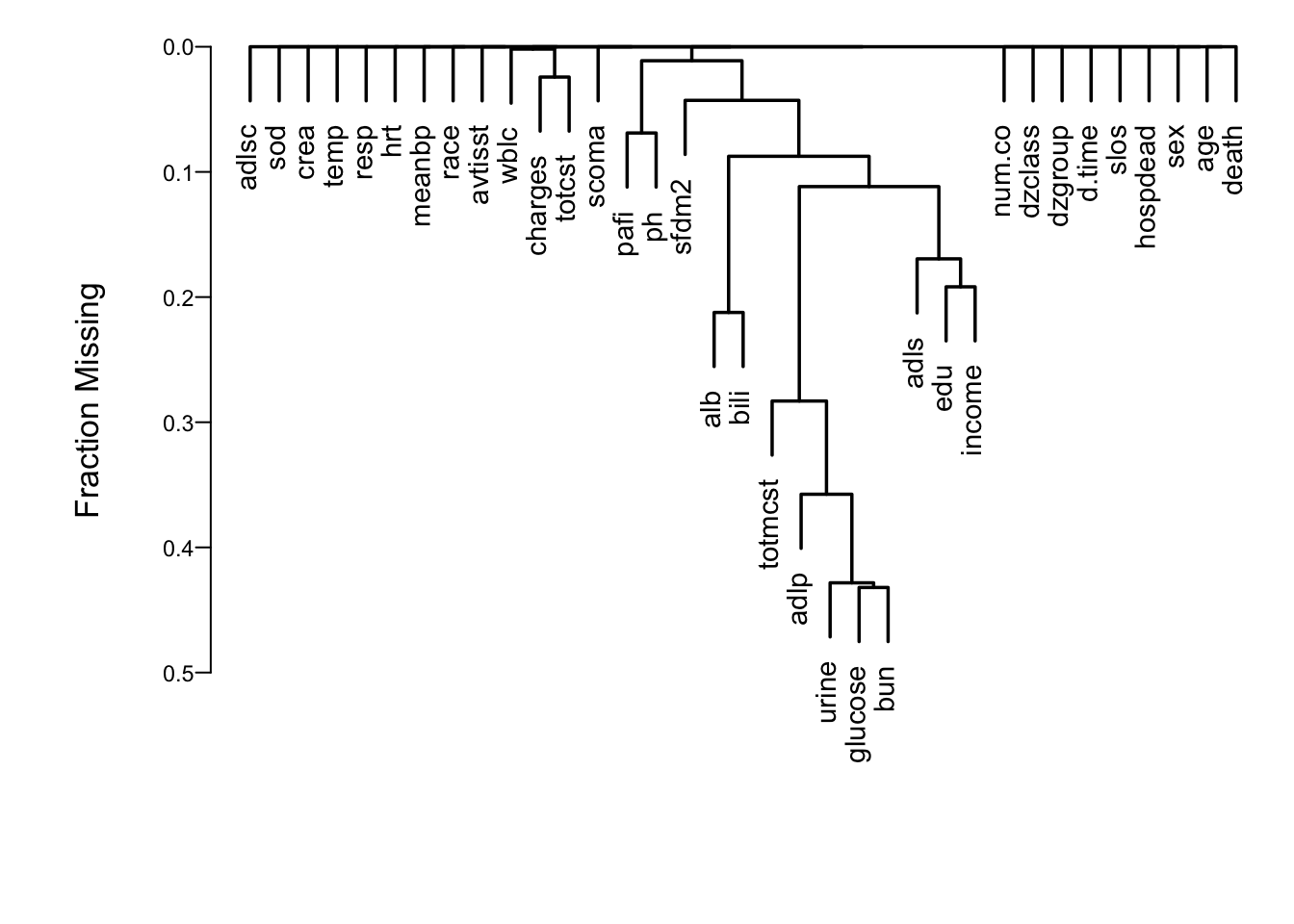Figure 19.1: Cluster analysis showing which predictors tend to be missing on the same patients Show associations between predictors using a general non-monotonic measure of dependence (Hoeffding $$D$$). C Code ac <- support[acute,] ac$dzgroup <- ac$dzgroup[drop=TRUE] # Remove unused levels vc <- varclus(~ age+sex+dzgroup+num.co+edu+income+scoma+race+ meanbp+wblc+hrt+resp+temp+pafi+alb+bili+crea+sod+ ph+glucose+bun+urine+adlsc, data=ac, sim='hoeffding') plot(vc)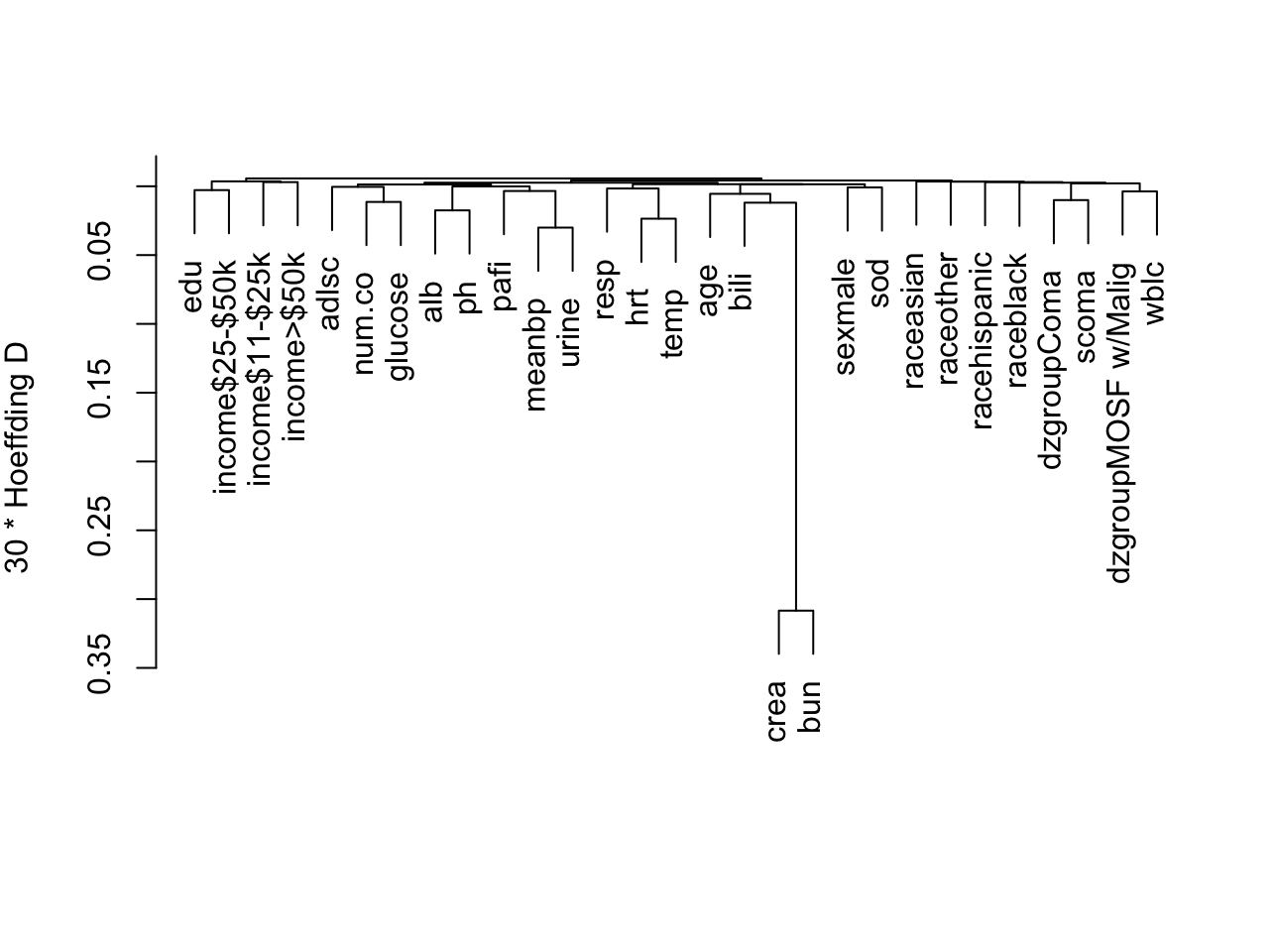Figure 19.2: Hierarchical clustering of potential predictors using Hoeffding $$D$$ as a similarity measure. Categorical predictors are automatically expanded into dummy variables. ## 19.2 Checking Adequacy of Log-Normal Accelerated Failure Time Model Code dd <- datadist(ac) # describe distributions of variables to rms options(datadist='dd') # Generate right-censored survival time variable ac <- upData(ac, print=FALSE, years = d.time/365.25, units = c(years = 'Year'), S = Surv(years, death)) # Show normal inverse Kaplan-Meier estimates # stratified by dzgroup survplot(npsurv(S ~ dzgroup, data=ac), conf='none', fun=qnorm,logt=TRUE)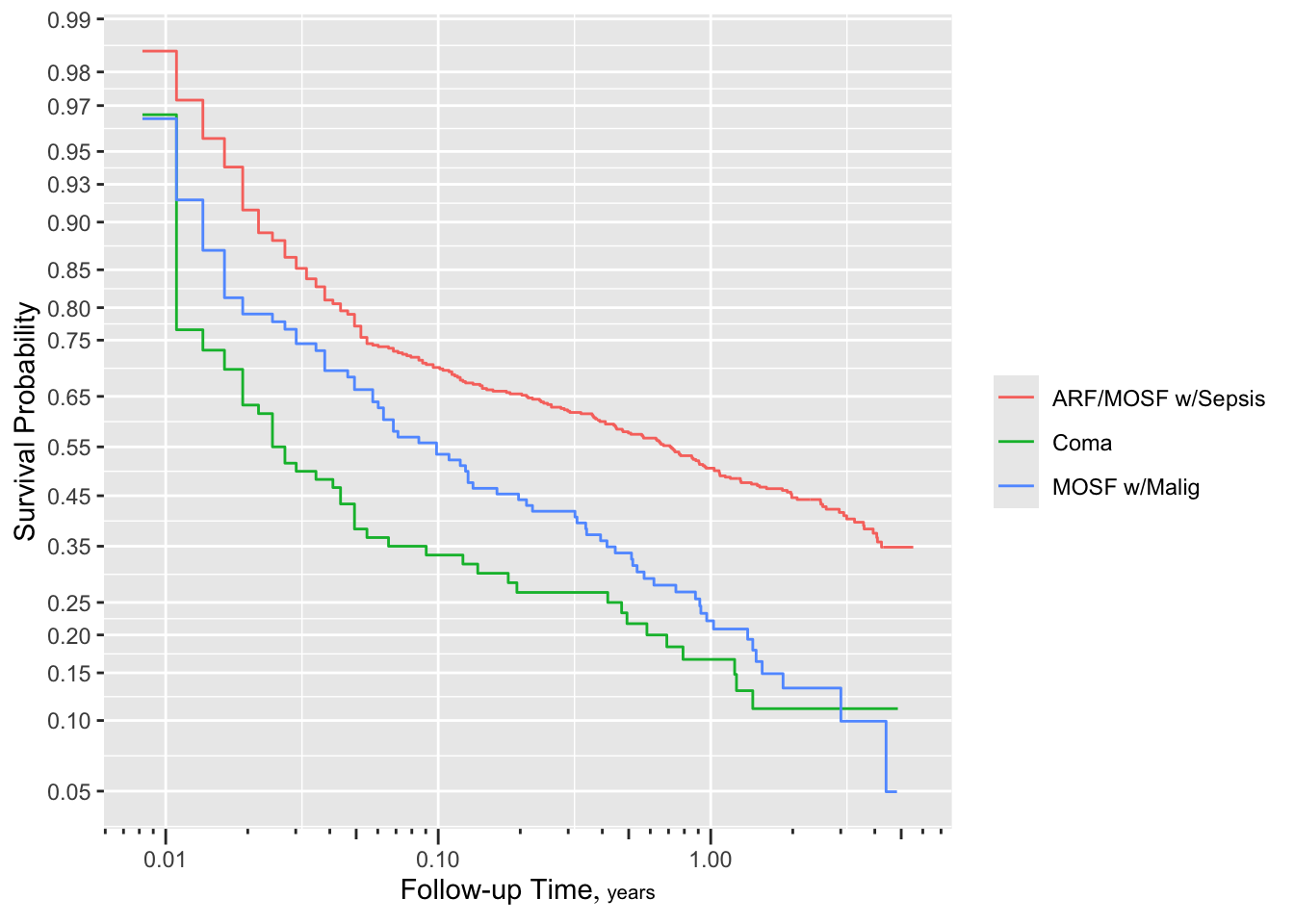Figure 19.3: $$\Phi^{-1}(S_{ ext{KM}}(t))$$ stratified by dzgroup. Linearity and semi-parallelism indicate a reasonable fit to the log-normal accelerated failure time model with respect to one predictor. More stringent assessment of log-normal assumptions: check distribution of residuals from an adjusted model: D Code spar(mfrow=c(2,2), ps=8, top=1, lwd=1) f <- psm(S ~ dzgroup + rcs(age,5) + rcs(meanbp,5), dist='lognormal', y=TRUE, data=ac) r <- resid(f) with(ac, { survplot(r, dzgroup, label.curve=FALSE) survplot(r, age, label.curve=FALSE) survplot(r, meanbp, label.curve=FALSE) survplot(r, runif(length(age)), label.curve=FALSE) } )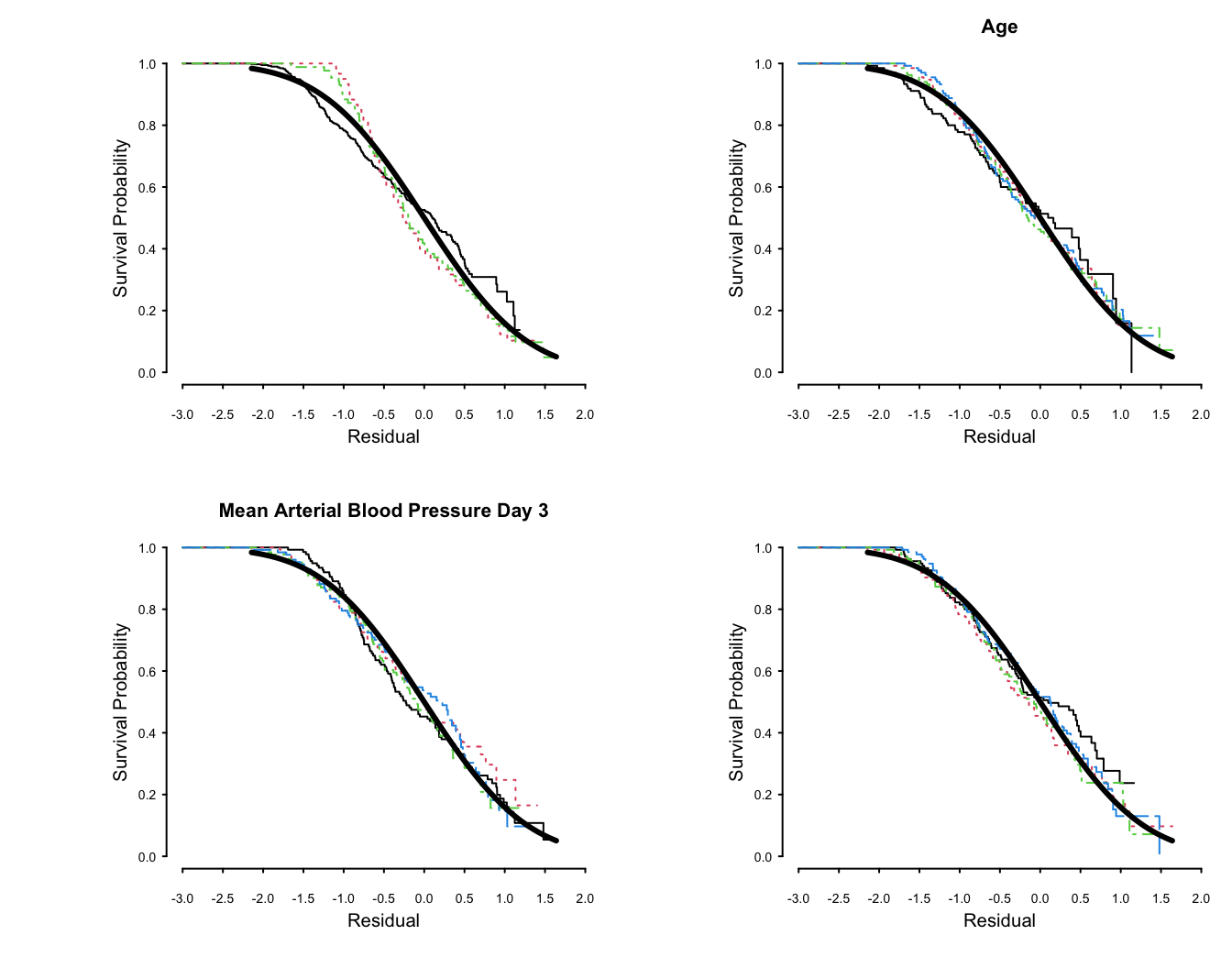Figure 19.4: Kaplan-Meier estimates of distributions of normalized, right-censored residuals from the fitted log-normal survival model. Residuals are stratified by important variables in the model (by quartiles of continuous variables), plus a random variable to depict the natural variability (in the lower right plot). Theoretical standard Gaussian distributions of residuals are shown with a thick solid line. The upper left plot is with respect to disease group. The fit for dzgroup is not great but overall fit is good. Remove from consideration predictors that are missing in $$> 0.2$$ of the patients. Many of these were only collected for the second phase of SUPPORT. Of those variables to be included in the model, find which ones have enough potential predictive power to justify allowing for nonlinear relationships or multiple categories, which spend more d.f. For each variable compute Spearman $$\rho^2$$ based on multiple linear regression of rank($$x$$), rank($$x$$)$$^2$$ and the survival time, truncating survival time at the shortest follow-up for survivors (356 days). This rids the data of censoring but creates many ties at 356 days. Code spar(top=1, ps=10, rt=3) ac <- upData(ac, print=FALSE, shortest.follow.up = min(d.time[death==0], na.rm=TRUE), d.timet = pmin(d.time, shortest.follow.up)) w <- spearman2(d.timet ~ age + num.co + scoma + meanbp + hrt + resp + temp + crea + sod + adlsc + wblc + pafi + ph + dzgroup + race, p=2, data=ac) plot(w, main='')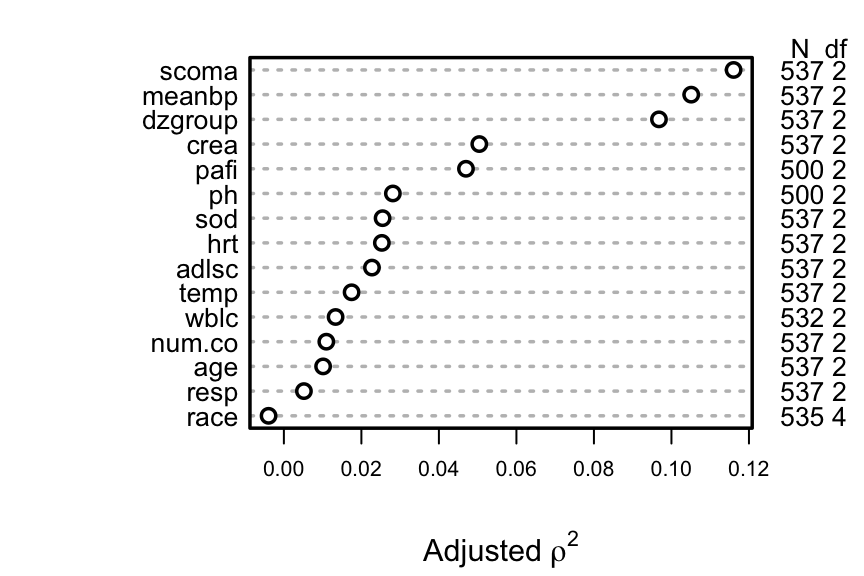Figure 19.5: Generalized Spearman $$\rho^2$$ rank correlation between predictors and truncated survival time A better approach is to use the complete information in the failure and censoring times by computing Somers’ $$D_{xy}$$ rank correlation allowing for censoring. E Code spar(top=1, ps=10, rt=3) w <- rcorrcens(S ~ age + num.co + scoma + meanbp + hrt + resp + temp + crea + sod + adlsc + wblc + pafi + ph + dzgroup + race, data=ac) plot(w, main='')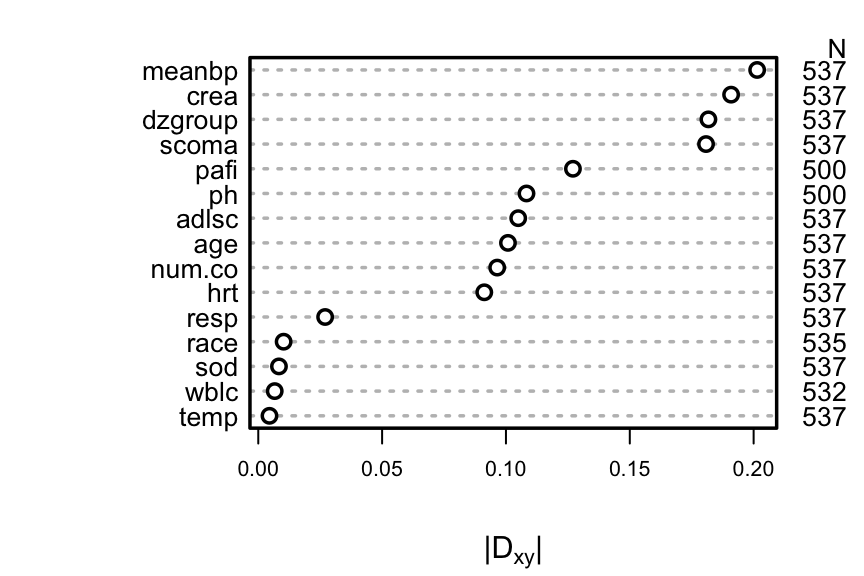Figure 19.6: Somers’ $$D_{xy}$$ rank correlation between predictors and original survival time. For dzgroup or race, the correlation coefficient is the maximum correlation from using a dummy variable to represent the most frequent or one to represent the second most frequent category. Code # Compute number of missing values per variable sapply(ac[.q(age,num.co,scoma,meanbp,hrt,resp,temp,crea,sod,adlsc, wblc,pafi,ph)], function(x) sum(is.na(x)))  age num.co scoma meanbp hrt resp temp crea sod adlsc wblc 0 0 0 0 0 0 0 0 0 0 5 pafi ph 37 37  Code # Can also do naplot(naclus(support[acute,])) # Can also use the Hmisc naclus and naplot functions to do this # Impute missing values with normal or modal values ac <- upData(ac, print=FALSE, wblc.i = impute(wblc, 9), pafi.i = impute(pafi, 333.3), ph.i = impute(ph, 7.4), race2 = ifelse(is.na(race), 'white', ifelse(race != 'white', 'other', 'white')) ) dd <- datadist(ac) Do a formal redundancy analysis using more than pairwise associations, and allow for non-monotonic transformations in predicting each predictor from all other predictors. This analysis requires missing values to be imputed so as to not greatly reduce the sample size. F Code redun(~ crea + age + sex + dzgroup + num.co + scoma + adlsc + race2 + meanbp + hrt + resp + temp + sod + wblc.i + pafi.i + ph.i, data=ac, nk=4)  Redundancy Analysis ~crea + age + sex + dzgroup + num.co + scoma + adlsc + race2 + meanbp + hrt + resp + temp + sod + wblc.i + pafi.i + ph.i n: 537 p: 16 nk: 4 Number of NAs: 0 Transformation of target variables forced to be linear R-squared cutoff: 0.9 Type: ordinary R^2 with which each variable can be predicted from all other variables: crea age sex dzgroup num.co scoma adlsc race2 meanbp hrt 0.133 0.246 0.132 0.451 0.147 0.418 0.153 0.151 0.178 0.258 resp temp sod wblc.i pafi.i ph.i 0.131 0.197 0.135 0.093 0.143 0.171 No redundant variables Better approach to gauging predictive potential and allocating d.f.: • Allow all continuous variables to have a the maximum number of knots entertained, in a log-normal survival model • Must use imputation to avoid losing data • Fit a “saturated” main effects model • Makes full use of censored data • Had to limit to 4 knots, force scoma to be linear, and omit ph.i to avoid singularity G Code k <- 4 f <- psm(S ~ rcs(age,k)+sex+dzgroup+pol(num.co,2)+scoma+ pol(adlsc,2)+race+rcs(meanbp,k)+rcs(hrt,k)+rcs(resp,k)+ rcs(temp,k)+rcs(crea,k)+rcs(sod,k)+rcs(wblc.i,k)+ rcs(pafi.i,k), dist='lognormal', data=ac, x=TRUE, y=TRUE) plot(anova(f, test='LR'))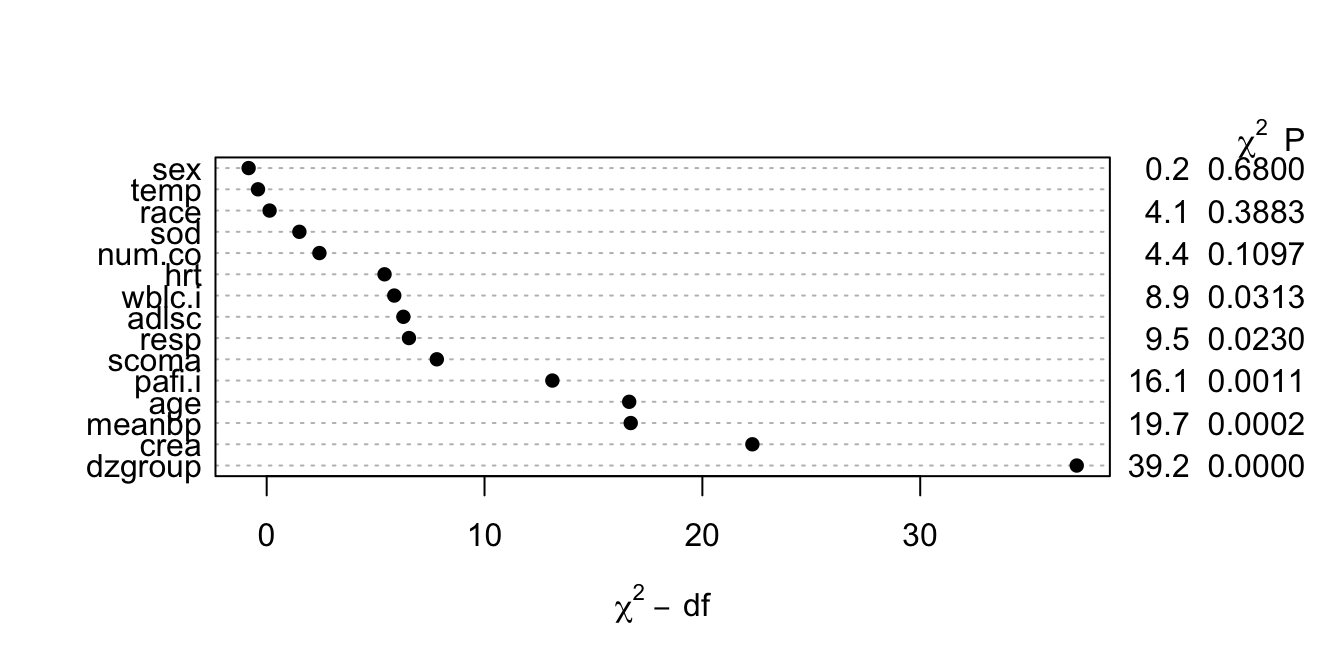Figure 19.7: Partial likelihood ratio $$\chi^{2}$$ statistics for association of each predictor with response from saturated main effects model, penalized for d.f. • This figure properly blinds the analyst to the form of effects (tests of linearity). • Fit a log-normal survival model with number of parameters corresponding to nonlinear effects determined from Figure 19.7. For the most promising predictors, five knots can be allocated, as there are fewer singularity problems once less promising predictors are simplified. HI Note: Since the audio was recorded, a bug in psm was fixed on 2017-03-12. Discrimination indexes shown in the table below are correct but the audio is incorrect for $$g$$ and $$g_{r}$$. Code f <- psm(S ~ rcs(age,5) + sex + dzgroup + num.co + scoma + pol(adlsc,2) + race2 + rcs(meanbp,5) + rcs(hrt,3) + rcs(resp,3) + temp + rcs(crea,4) + sod + rcs(wblc.i,3) + rcs(pafi.i,4), dist='lognormal', data=ac, x=TRUE, y=TRUE) f Parametric Survival Model: Log Normal Distribution psm(formula = S ~ rcs(age, 5) + sex + dzgroup + num.co + scoma + pol(adlsc, 2) + race2 + rcs(meanbp, 5) + rcs(hrt, 3) + rcs(resp, 3) + temp + rcs(crea, 4) + sod + rcs(wblc.i, 3) + rcs(pafi.i, 4), data = ac, dist = "lognormal", x = TRUE, y = TRUE)  Model Likelihood Ratio Test Discrimination Indexes Obs 537 LR χ2 236.83 R2 0.594 Events 356 d.f. 30 R230,537 0.320 σ 2.2308 Pr(>χ2) <0.0001 R230,356 0.441 Dxy 0.485 β S.E. Wald Z Pr(>|Z|) (Intercept) -5.3904 3.7603 -1.43 0.1517 age -0.0148 0.0309 -0.48 0.6322 age' -0.0412 0.1078 -0.38 0.7024 age'' 0.1670 0.5594 0.30 0.7653 age''' -0.2099 1.3707 -0.15 0.8783 sex=male -0.0737 0.2181 -0.34 0.7354 dzgroup=Coma -2.0676 0.4062 -5.09 <0.0001 dzgroup=MOSF w/Malig -1.4664 0.3112 -4.71 <0.0001 num.co -0.1917 0.0858 -2.23 0.0255 scoma -0.0142 0.0044 -3.25 0.0011 adlsc -0.3735 0.1520 -2.46 0.0140 adlsc2 0.0442 0.0243 1.82 0.0691 race2=white -0.2979 0.2658 -1.12 0.2624 meanbp 0.0702 0.0210 3.34 0.0008 meanbp' -0.3080 0.2261 -1.36 0.1732 meanbp'' 0.8438 0.8556 0.99 0.3241 meanbp''' -0.5715 0.7707 -0.74 0.4584 hrt -0.0171 0.0069 -2.46 0.0140 hrt' 0.0064 0.0063 1.02 0.3090 resp 0.0454 0.0230 1.97 0.0483 resp' -0.0851 0.0291 -2.93 0.0034 temp 0.0523 0.0834 0.63 0.5308 crea -0.4585 0.6727 -0.68 0.4955 crea' -11.5176 19.0027 -0.61 0.5444 crea'' 21.9840 31.0113 0.71 0.4784 sod 0.0044 0.0157 0.28 0.7792 wblc.i 0.0746 0.0331 2.25 0.0242 wblc.i' -0.0880 0.0377 -2.34 0.0195 pafi.i 0.0169 0.0055 3.07 0.0021 pafi.i' -0.0569 0.0239 -2.38 0.0173 pafi.i'' 0.1088 0.0482 2.26 0.0239 Log(scale) 0.8024 0.0401 19.99 <0.0001 Code a <- anova(f, test='LR') ## 19.3 Summarizing the Fitted Model • Plot the shape of the effect of each predictor on log survival time. • All effects centered: can be placed on common scale • LR $$\chi^2$$ statistics, penalized for d.f., plotted in descending order J Code ggplot(Predict(f, ref.zero=TRUE), vnames='names', sepdiscrete='vertical', anova=a)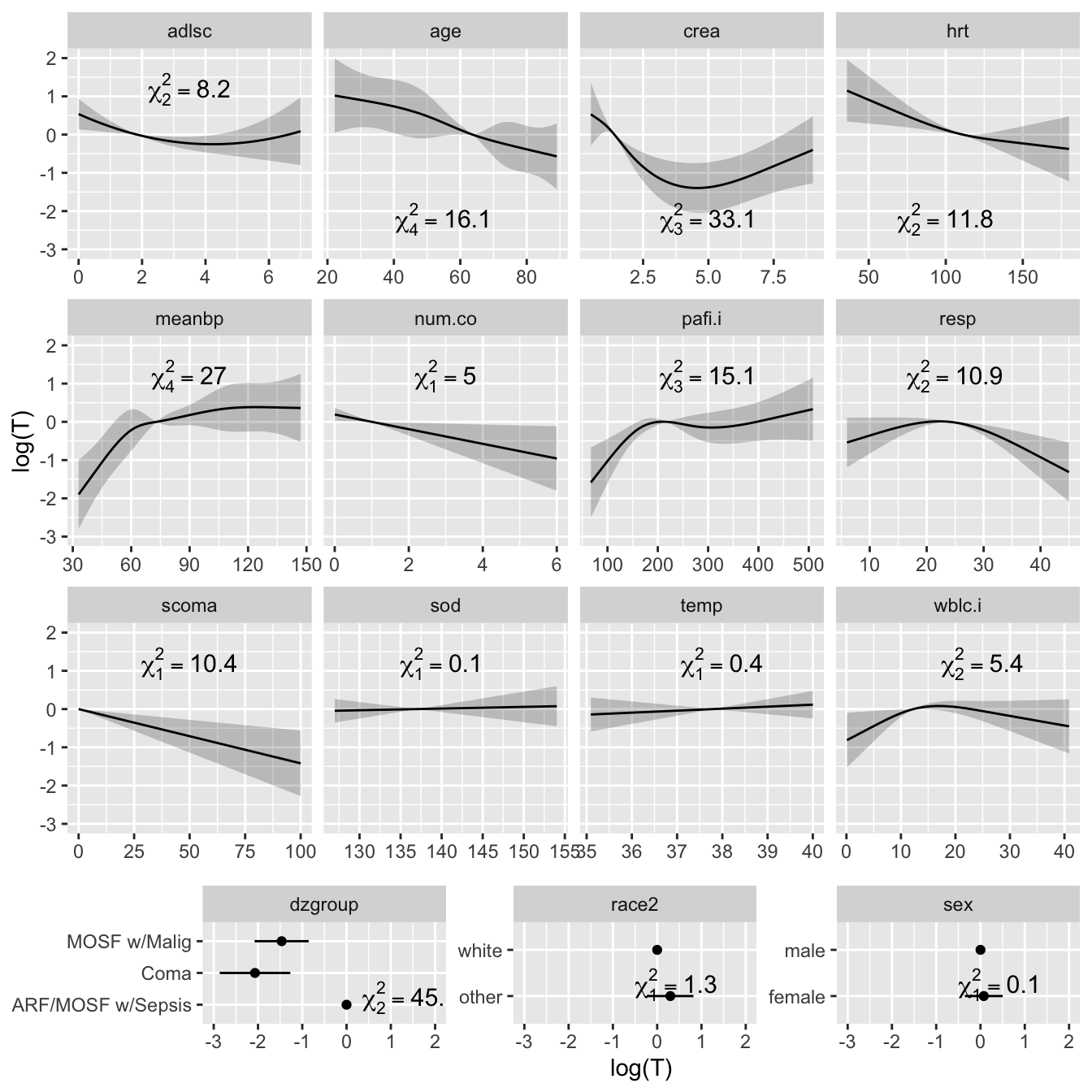Figure 19.8: Effect of each predictor on log survival time. Predicted values have been centered so that predictions at predictor reference values are zero. Pointwise 0.95 confidence bands are also shown. As all $$Y$$-axes have the same scale, it is easy to see which predictors are strongest. K Code a Likelihood Ratio Statistics for S χ2 d.f. P age 16.10 4 0.0029 Nonlinear 0.23 3 0.9722 sex 0.11 1 0.7354 dzgroup 45.11 2 <0.0001 num.co 5.00 1 0.0253 scoma 10.44 1 0.0012 adlsc 8.24 2 0.0162 Nonlinear 3.30 1 0.0694 race2 1.26 1 0.2618 meanbp 26.99 4 <0.0001 Nonlinear 10.38 3 0.0156 hrt 11.82 2 0.0027 Nonlinear 1.04 1 0.3079 resp 10.94 2 0.0042 Nonlinear 8.49 1 0.0036 temp 0.39 1 0.5309 crea 33.14 3 <0.0001 Nonlinear 21.05 2 <0.0001 sod 0.08 1 0.7792 wblc.i 5.44 2 0.0659 Nonlinear 5.43 1 0.0198 pafi.i 15.13 3 0.0017 Nonlinear 6.93 2 0.0312 TOTAL NONLINEAR 58.28 14 <0.0001 TOTAL 236.83 30 <0.0001 Code plot(a)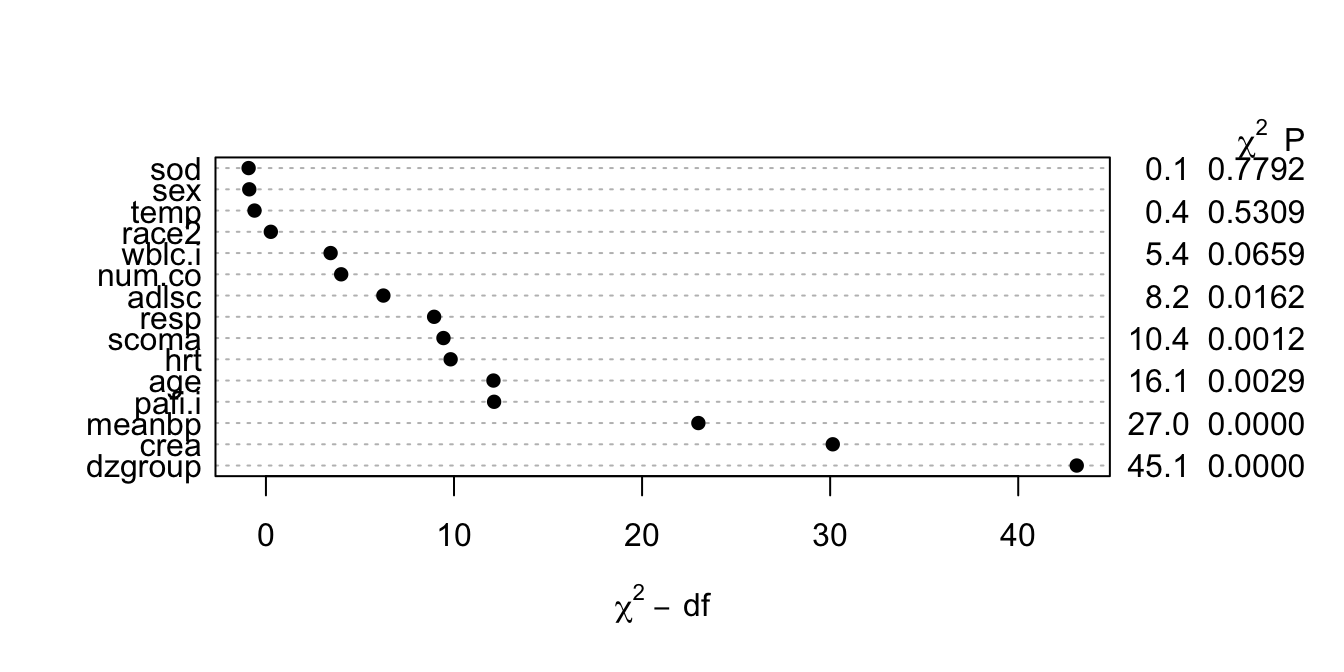Figure 19.9: Contribution of variables in predicting survival time in log-normal model L Code spar(top=1, ps=11) options(digits=3) plot(summary(f), log=TRUE, main='')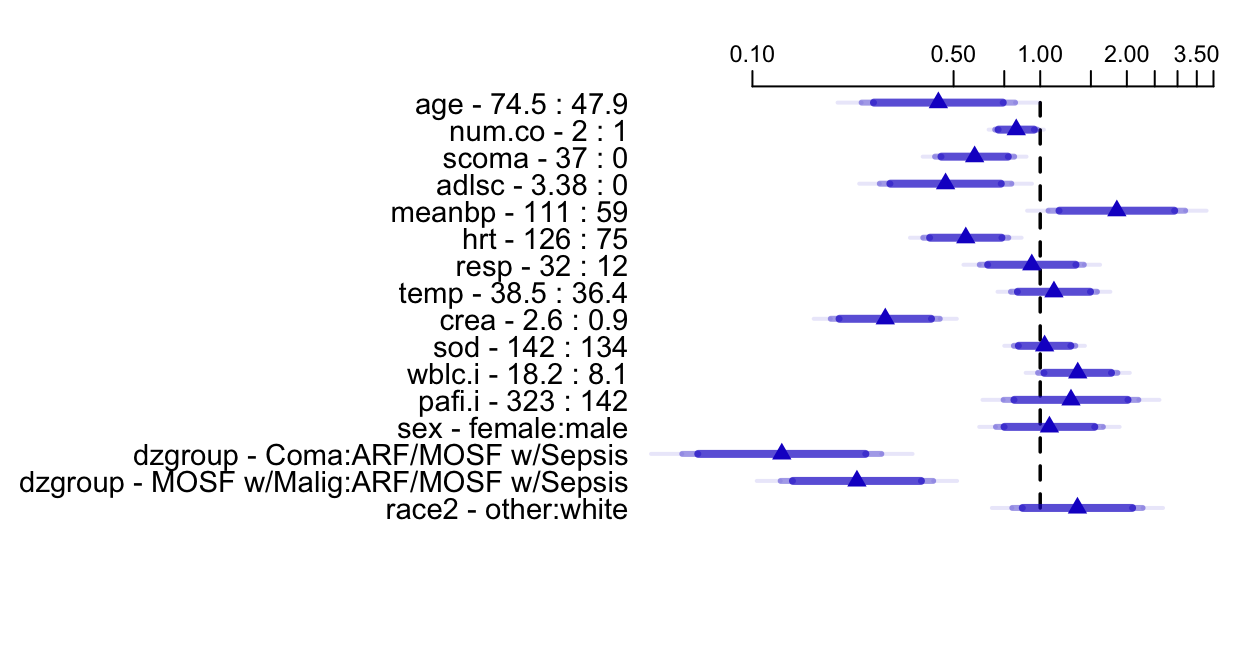Figure 19.10: Estimated survival time ratios for default settings of predictors. For example, when age changes from its lower quartile to the upper quartile (47.9y to 74.5y), median survival time decreases by more than half. Different shaded areas of bars indicate different confidence levels (0.9, 0.95, 0.99). ## 19.4 Internal Validation of the Fitted Model Using the Bootstrap Validate indexes describing the fitted model. Code # First add data to model fit so bootstrap can re-sample # from the data g <- update(f, x=TRUE, y=TRUE) set.seed(717) validate(g, B=300, dxy=TRUE) Index Original Sample Training Sample Test Sample Optimism Corrected Index Successful Resamples Dxy 0.4852 0.5099 0.4593 0.0506 0.4346 300 R2 0.594 0.6582 0.5383 0.1199 0.4741 300 Intercept 0 0 -0.0454 0.0454 -0.0454 300 Slope 1 1 0.9057 0.0943 0.9057 300 D 0.4788 0.5488 0.4237 0.1251 0.3537 300 U -0.0041 -0.0041 -0.0097 0.0056 -0.0096 300 Q 0.4829 0.553 0.4334 0.1196 0.3633 300 g 1.9593 2.0516 1.8684 0.1832 1.7761 300 • From $$D_{xy}$$ and $$R^2$$ there is a moderate amount of overfitting. • Slope shrinkage factor (0.90) is not troublesome • Almost unbiased estimate of future predictive discrimination on similar patients is the corrected $$D_{xy}$$ of 0.43. M Validate predicted 1-year survival probabilities. Use a smooth approach that does not require binning and use less precise Kaplan-Meier estimates obtained by stratifying patients by the predicted probability, with at least 60 patients per group. N Code set.seed(717) cal <- calibrate(g, u=1, B=300) plot(cal, subtitles=FALSE) cal <- calibrate(g, cmethod='KM', u=1, m=60, B=300, pr=FALSE) plot(cal, add=TRUE)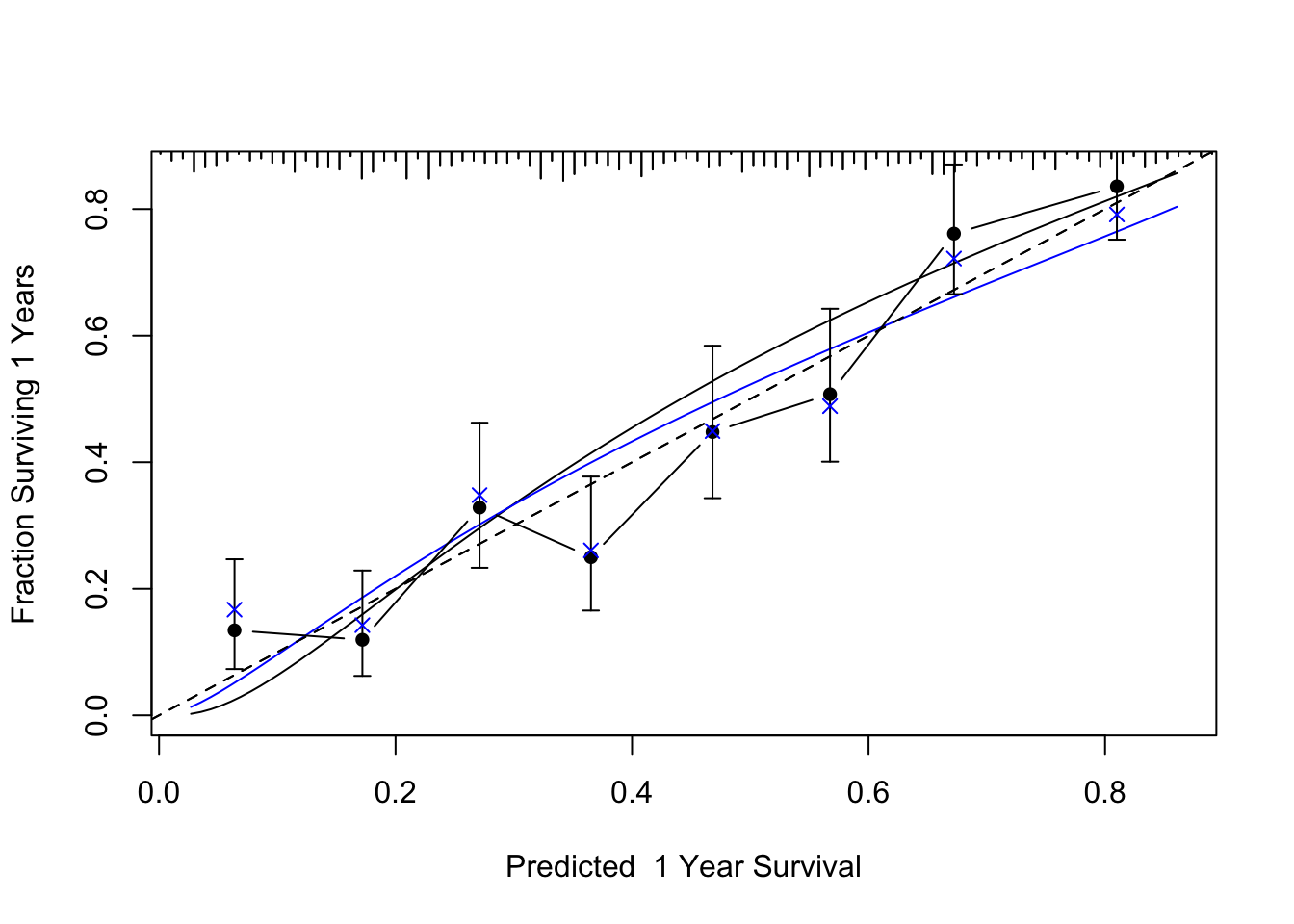Figure 19.11: Bootstrap validation of calibration curve. Dots represent apparent calibration accuracy; $$\times$$ are bootstrap estimates corrected for overfitting, based on binning predicted survival probabilities and and computing Kaplan-Meier estimates. Black curve is the estimated observed relationship using hare and the blue curve is the overfitting-corrected hare estimate. The gray-scale line depicts the ideal relationship. ## 19.5 Approximating the Full Model The fitted log-normal model is perhaps too complex for routine use and for routine data collection. Let us develop a simplified model that can predict the predicted values of the full model with high accuracy ($$R^{2} = 0.96$$). The simplification is done using a fast backward stepdown against the full model predicted values. Code Z <- predict(f) # X*beta hat a <- ols(Z ~ rcs(age,5)+sex+dzgroup+num.co+ scoma+pol(adlsc,2)+race2+ rcs(meanbp,5)+rcs(hrt,3)+rcs(resp,3)+ temp+rcs(crea,4)+sod+rcs(wblc.i,3)+ rcs(pafi.i,4), sigma=1, data=ac) # sigma=1 is used to prevent sigma hat from being zero when # R2=1.0 since we start out by approximating Z with all # component variables fastbw(a, aics=10000) # fast backward stepdown  Deleted Chi-Sq d.f. P Residual d.f. P AIC R2 sod 0.43 1 0.512 0.43 1 0.5117 -1.57 1.000 sex 0.57 1 0.451 1.00 2 0.6073 -3.00 0.999 temp 2.20 1 0.138 3.20 3 0.3621 -2.80 0.998 race2 6.81 1 0.009 10.01 4 0.0402 2.01 0.994 wblc.i 29.52 2 0.000 39.53 6 0.0000 27.53 0.976 num.co 30.84 1 0.000 70.36 7 0.0000 56.36 0.957 resp 54.18 2 0.000 124.55 9 0.0000 106.55 0.924 adlsc 52.46 2 0.000 177.00 11 0.0000 155.00 0.892 pafi.i 66.78 3 0.000 243.79 14 0.0000 215.79 0.851 scoma 78.07 1 0.000 321.86 15 0.0000 291.86 0.803 hrt 83.17 2 0.000 405.02 17 0.0000 371.02 0.752 age 68.08 4 0.000 473.10 21 0.0000 431.10 0.710 crea 314.47 3 0.000 787.57 24 0.0000 739.57 0.517 meanbp 403.04 4 0.000 1190.61 28 0.0000 1134.61 0.270 dzgroup 441.28 2 0.000 1631.89 30 0.0000 1571.89 0.000 Approximate Estimates after Deleting Factors Coef S.E. Wald Z P [1,] -0.5928 0.04315 -13.74 0 Factors in Final Model None Code f.approx <- ols(Z ~ dzgroup + rcs(meanbp,5) + rcs(crea,4) + rcs(age,5) + rcs(hrt,3) + scoma + rcs(pafi.i,4) + pol(adlsc,2)+ rcs(resp,3), x=TRUE, data=ac) f.approx$stats
         n Model L.R.       d.f.         R2          g      Sigma
537.000   1688.225     23.000      0.957      1.915      0.370 
• Estimate variance-covariance matrix of the coefficients of reduced model
• This covariance matrix does not include the scale parameter
O
Code
V <- vcov(f, regcoef.only=TRUE)     # var(full model)
X <- cbind(Intercept=1, g$x) # full model design x <- cbind(Intercept=1, f.approx$x) # approx. model design
w <- solve(t(x) %*% x, t(x)) %*% X  # contrast matrix
v <- w %*% V %*% t(w)

Compare variance estimates (diagonals of v) with variance estimates from a reduced model that is fitted against the actual outcomes.

Code
f.sub <- psm(S ~ dzgroup + rcs(meanbp,5) + rcs(crea,4) + rcs(age,5) +
rcs(hrt,3) + scoma + rcs(pafi.i,4) + pol(adlsc,2)+
rcs(resp,3), dist='lognormal', data=ac)

r <- diag(v)/diag(vcov(f.sub,regcoef.only=TRUE))
r[c(which.min(r), which.max(r))]
 hrt'   age
0.976 0.982 

P
Code
f.approx\$var <- v
anova(f.approx, test='Chisq', ss=FALSE)
Wald Statistics for Z
χ2 d.f. P
dzgroup 55.94 2 <0.0001
meanbp 29.87 4 <0.0001
Nonlinear 9.84 3 0.0200
crea 39.04 3 <0.0001
Nonlinear 24.37 2 <0.0001
age 18.12 4 0.0012
Nonlinear 0.34 3 0.9517
hrt 9.87 2 0.0072
Nonlinear 0.40 1 0.5289
scoma 9.85 1 0.0017
pafi.i 14.01 3 0.0029
Nonlinear 6.66 2 0.0357
Nonlinear 2.87 1 0.0904
resp 9.65 2 0.0080
Nonlinear 7.13 1 0.0076
TOTAL NONLINEAR 58.08 13 <0.0001
TOTAL 252.32 23 <0.0001

Equation for simplified model:

Code
# Typeset mathematical form of approximate model
latex(f.approx)
$\mathrm{E}(\mathrm{Z}) = X\beta,~~\mathrm{where}$ $\begin{array} \lefteqn{X\hat{\beta}=}\\ & & -2.51 \\ & & -1.94[\mathrm{Coma}]-1.75[\mathrm{MOSF\ w/Malig}] \\ & & + 0.068 \mathrm{meanbp}-3.08\!\times\!10^{-5}(\mathrm{meanbp}-41.8)_{+}^{3}+7.9\!\times\!10^{-5 }(\mathrm{meanbp}-61)_{+}^{3} \\ & & -4.91\!\times\!10^{-5}(\mathrm{meanbp}-73)_{+}^{3}+2.61\!\times\!10^{-6 }(\mathrm{meanbp}-109)_{+}^{3}-1.7\!\times\!10^{-6 }(\mathrm{meanbp}-135)_{+}^{3} \\ & & -0.553\mathrm{crea}-0.229(\mathrm{crea}-0.6)_{+}^{3}+0.45 (\mathrm{crea}-1.1)_{+}^{3}-0.233(\mathrm{crea}-1.94)_{+}^{3} \\ & & +0.0131(\mathrm{crea}-7.32)_{+}^{3} \\ & & -0.0165 \mathrm{age}-1.13\!\times\!10^{-5}(\mathrm{age}-28.5)_{+}^{3}+4.05\!\times\!10^{-5 }(\mathrm{age}-49.5)_{+}^{3} \\ & & -2.15\!\times\!10^{-5}(\mathrm{age}-63.7)_{+}^{3}-2.68\!\times\!10^{-5}(\mathrm{age}-72.7)_{+}^{3}+1.9\!\times\!10^{-5 }(\mathrm{age}-85.6)_{+}^{3} \\ & & -0.0136 \mathrm{hrt}+6.09\!\times\!10^{-7 }(\mathrm{hrt}-60)_{+}^{3}-1.68\!\times\!10^{-6}(\mathrm{hrt}-111)_{+}^{3}+1.07\!\times\!10^{-6 }(\mathrm{hrt}-140)_{+}^{3} \\ & & -0.0135\:\mathrm{scoma} \\ & & + 0.0161 \mathrm{pafi.i}-4.77\!\times\!10^{-7}(\mathrm{pafi.i}-88)_{+}^{3}+9.11\!\times\!10^{-7 }(\mathrm{pafi.i}-167)_{+}^{3} \\ & & -5.02\!\times\!10^{-7}(\mathrm{pafi.i}-276)_{+}^{3}+6.76\!\times\!10^{-8 }(\mathrm{pafi.i}-426)_{+}^{3} -0.369\:\mathrm{adlsc}+0.0409\:\mathrm{adlsc}^{2} \\ & & + 0.0394 \mathrm{resp}-9.11\!\times\!10^{-5}(\mathrm{resp}-10)_{+}^{3}+0.000176 (\mathrm{resp}-24)_{+}^{3}-8.5\!\times\!10^{-5 }(\mathrm{resp}-39)_{+}^{3} \\ \end{array}$

$[c]=1~\mathrm{if~subject~is~in~group}~c,~0~\mathrm{otherwise}$$(x)_{+}=x~\mathrm{if}~x > 0,~0~\mathrm{otherwise}$

Nomogram for predicting median and mean survival time, based on approximate model:

Code
# Derive R functions that express mean and quantiles
# of survival time for specific linear predictors
# analytically
expected.surv <- Mean(f)
quantile.surv <- Quantile(f)
expected.surv
function (lp = NULL, parms = 0.802352037606488)
{
names(parms) <- NULL
exp(lp + exp(2 * parms)/2)
}
<environment: namespace:rms>
Code
quantile.surv
function (q = 0.5, lp = NULL, parms = 0.802352037606488)
{
names(parms) <- NULL
f <- function(lp, q, parms) lp + exp(parms) * qnorm(q)
names(q) <- format(q)
drop(exp(outer(lp, q, FUN = f, parms = parms)))
}
<environment: namespace:rms>
Code
median.surv   <- function(x) quantile.surv(lp=x)
Code
spar(ps=10)
# Improve variable labels for the nomogram
f.approx <- Newlabels(f.approx, c('Disease Group','Mean Arterial BP',
'Creatinine','Age','Heart Rate','SUPPORT Coma Score',
nom <-
nomogram(f.approx,
pafi.i=c(0, 50, 100, 200, 300, 500, 600, 700, 800, 900),
fun=list('Median Survival Time'=median.surv,
'Mean Survival Time'  =expected.surv),
fun.at=c(.1,.25,.5,1,2,5,10,20,40))
plot(nom, cex.var=1, cex.axis=.75, lmgp=.25)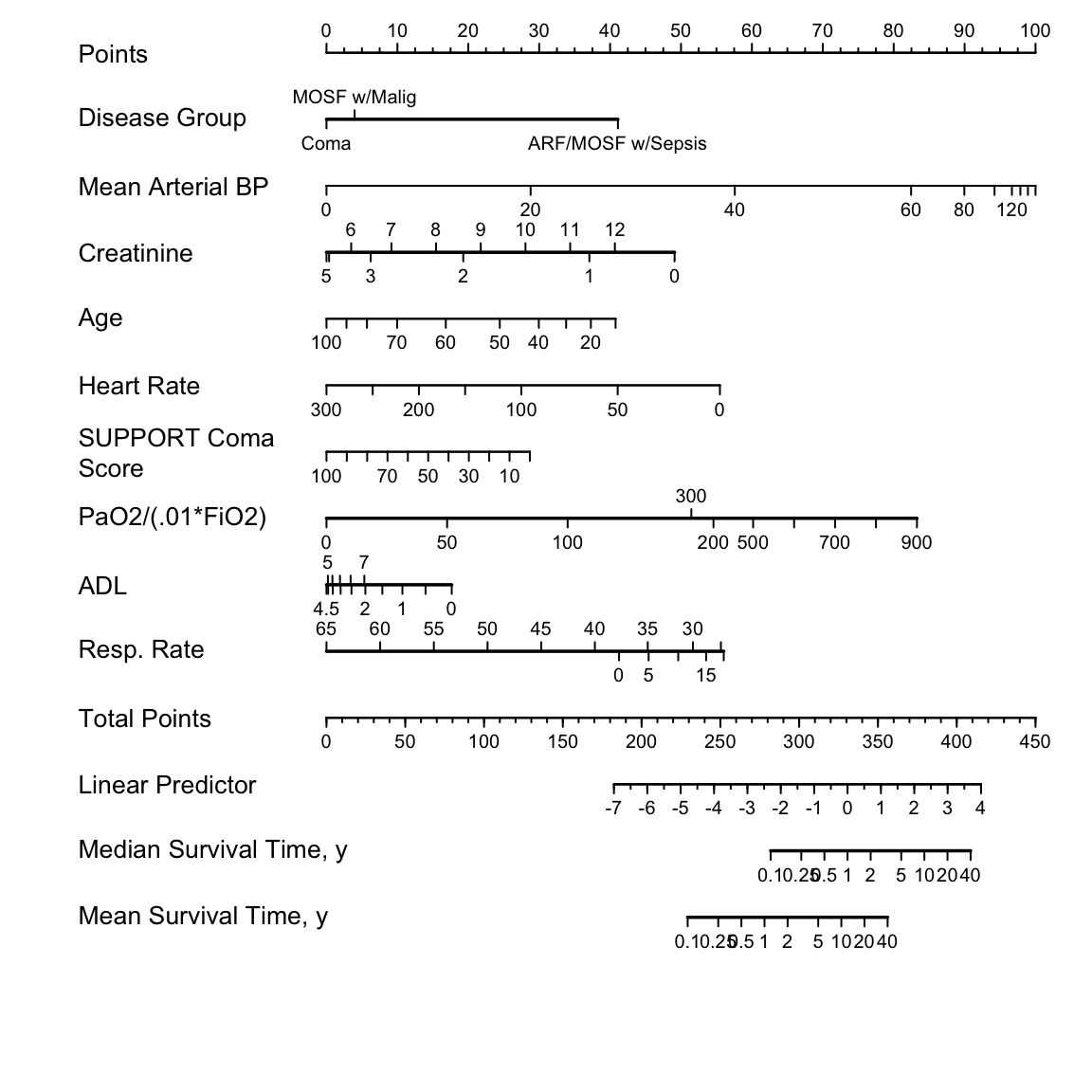Figure 19.12: Nomogram for predicting median and mean survival time, based on approximation of full model
R packages and functions used. All packages are available on CRAN.
Packages Purpose Functions
Hmisc Miscellaneous functions describe,ecdf,naclus,varclus,llist,spearman2,impute,latex
rms Modeling datadist,psm,rcs,ols,fastbw
Model presentation survplot,Newlabels,Function,Mean,Quantile,nomogram
Model validation validate,calibrate